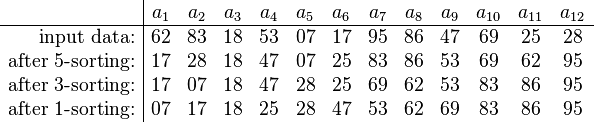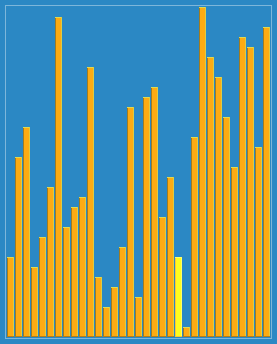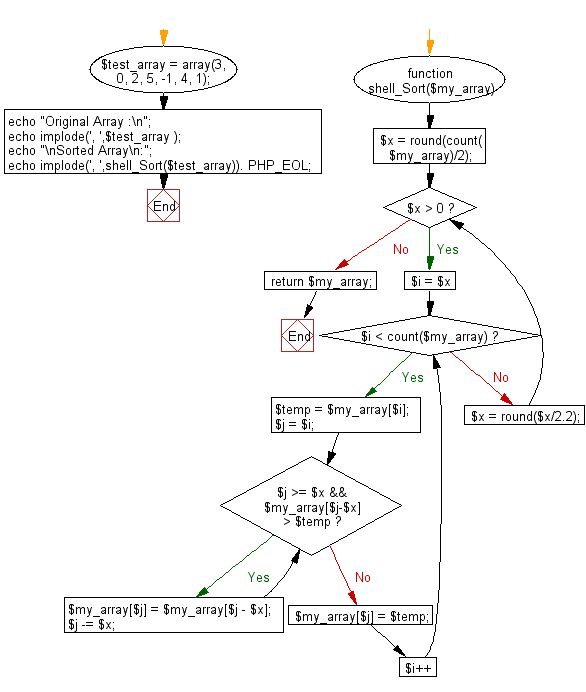﻿ PHP: Sort a list of elements using Shell sort - w3resource

# PHP Searching and Sorting Algorithm: Shell sort

## PHP Searching and Sorting Algorithm: Exercise-5 with Solution

Write a PHP program to sort a list of elements using Shell sort.

According to Wikipedia "Shell sort or Shell's method, is an in-place comparison sort. It can be seen as either a generalization of sorting by exchange (bubble sort) or sorting by insertion (insertion sort). The method starts by sorting pairs of elements far apart from each other, then progressively reducing the gap between elements to be compared. Starting with far apart elements can move some out-of-place elements into position faster than a simple nearest neighbor exchange."

An example run of Shellsort with gaps 5, 3 and 1 is shown below.The first pass, 5-sorting, performs insertion sort on separate subarrays (a1, a6, a11), (a2, a7, a12), (a3, a8), (a4, a9), (a5, a10). For instance, it changes the subarray (a1, a6, a11) from (62, 17, 25) to (17, 25, 62). The next pass, 3-sorting, performs insertion sort on the subarrays (a1, a4, a7, a10), (a2, a5, a8, a11), (a3, a6, a9, a12). The last pass, 1-sorting, is an ordinary insertion sort of the entire array (a1,..., a12). As the example illustrates, the subarrays that Shellsort operates on are initially short; later they are longer but almost ordered. In both cases insertion sort works efficiently. Shellsort is unstable: it may change the relative order of elements with equal values. It is an adaptive sorting algorithm in that it executes faster when the input is partially sorted.

Shell SortShellsort with gaps 23, 10, 4, 1 in action.

Sample Solution :

PHP Code :

``````<?php
function shell_Sort(\$my_array)
{
\$x = round(count(\$my_array)/2);
while(\$x > 0)
{
for(\$i = \$x; \$i < count(\$my_array);\$i++){
\$temp = \$my_array[\$i];
\$j = \$i;
while(\$j >= \$x && \$my_array[\$j-\$x] > \$temp)
{
\$my_array[\$j] = \$my_array[\$j - \$x];
\$j -= \$x;
}
\$my_array[\$j] = \$temp;
}
\$x = round(\$x/2.2);
}
return \$my_array;
}

\$test_array = array(3, 0, 2, 5, -1, 4, 1);
echo "Original Array :\n";
echo implode(', ',\$test_array );
echo "\nSorted Array\n:";
echo implode(', ',shell_Sort(\$test_array)). PHP_EOL;
?>
```
```

Sample Output:

```Original Array :
3, 0, 2, 5, -1, 4, 1
Sorted Array :
-1, 0, 1, 2, 3, 4, 5
```

Flowchart :PHP Code Editor:

Have another way to solve this solution? Contribute your code (and comments) through Disqus.

What is the difficulty level of this exercise?

Test your Programming skills with w3resource's quiz.

﻿